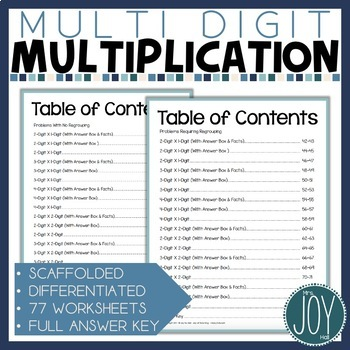# Multi Digit Multiplication Worksheets with 2 Digit by 2 Digit Multiplication4th - 5th
Subjects
Standards
Resource Type
Formats Included
• PDF
• EBooks
Pages

#### Also included in

1. Easy-to-implement differentiated math kits are quick and effective for teaching rules of divisibility, least common multiples, long division, coordinate graphing, decimals, probability, square roots, multi-digit multiplication, and MORE! Use these fifteen engaging math kits to captivate your student
\$86.45
\$123.50
Save \$37.05

### Description

Teaching multi-digit multiplication to students who don't know their multiplication facts? I have the perfect solution! Your students will quickly go from solving 2 x 1 digit multiplication problems to mastering 2 x 2 digit multiplication with this easy to use resource.

Be sure to click on the preview above to check out this wonderful teaching tool!

Also, follow me and be notified when new products are uploaded. New products are always 50% off for the first 24 hours they are posted. It pays to follow me!

Start with 2 x 1 digit multiplication worksheets with no regrouping that has needed multiplication facts listed at the bottom of the page. Students progress all the way through 4 x 2 digit multiplication with regrouping with no multiplication facts on the page. All problems are on grid paper, giving beginning or struggling students much needed help with spacing and number placement.

Differentiation is a breeze with these included skills:

• 2 x 1 digit multiplication without regrouping with multiplication facts listed
• 3 x 1 digit multiplication without regrouping with multiplication facts listed
• 4 x 1 digit multiplication without regrouping with multiplication facts listed
• 2 x 1 digit multiplication without regrouping
• 3 x 1 digit multiplication without regrouping
• 4 x 1 digit multiplication without regrouping
• 2 x 1 digit multiplication with regrouping with multiplication facts listed
• 3 x 1 digit multiplication with regrouping with multiplication facts listed
• 4 x 1 digit multiplication with regrouping with multiplication facts listed
• 2 x 1 digit multiplication with regrouping
• 3 x 1 digit multiplication with regrouping
• 4 x 1 digit multiplication with regrouping
• 2 x 2 digit multiplication without regrouping with multiplication facts listed
• 3 x 2 digit multiplication without regrouping with multiplication facts listed
• 4 x 2 digit multiplication without regrouping with multiplication facts listed
• 2 x 2 digit multiplication without regrouping
• 3 x 2 digit multiplication without regrouping
• 4 x 2 digit multiplication without regrouping
• 2 x 2 digit multiplication with regrouping with multiplication facts listed
• 3 x 2 digit multiplication with regrouping with multiplication facts listed
• 4 x 2 digit multiplication with regrouping with multiplication facts listed
• 2 x 2 digit multiplication with regrouping
• 3 x 2 digit multiplication with regrouping
• 4 x 2 digit multiplication with regrouping

This now includes options for scaffolding and error-less learning so that all students can be successful!

ALL problems are on grids. There are pages with the correct answers at the bottom as well as the multiplication facts required to complete the problems on that particular page.

*********************************************************

OTHER RESOURCES

Beginning Long Division Kit

Differentiated Least Common Multiples Pack

Perfect Squares and Square Roots Exploration Kit

*********************************************************

CONTACT ME!

Come and visit me at my Teachers Pay Teachers store!

Thank you!

Joy

Total Pages
Included
Teaching Duration
3 Weeks
Report this Resource to TpT
Reported resources will be reviewed by our team. Report this resource to let us know if this resource violates TpT’s content guidelines.

### Standards

to see state-specific standards (only available in the US).
Fluently multiply and divide within 100, using strategies such as the relationship between multiplication and division (e.g., knowing that 8 × 5 = 40, one knows 40 ÷ 5 = 8) or properties of operations. By the end of Grade 3, know from memory all products of two one-digit numbers.
Fluently multiply multi-digit whole numbers using the standard algorithm.
Multiply a whole number of up to four digits by a one-digit whole number, and multiply two two-digit numbers, using strategies based on place value and the properties of operations. Illustrate and explain the calculation by using equations, rectangular arrays, and/or area models.